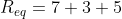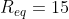# High School Physics : Understanding Series and Parallel

## Example Questions

### Example Question #1 : Understanding Series And Parallel

Each branch of a parallel circuit carries an equal __________.

capacitance

resistance

voltage

power

current

voltage

Explanation:

By definition, each branch of a parallel circuit will have the same voltage. The current, however, may be split between branches in inequal amounts. In contrast, a series circuit will carry a constant current throughout the circuit, but voltage may differ between elements.

Resistance and capacitance are dependent on the elements present in a given circuit, so no claims can be made. Power is similarly dependent on the elements present in a specific circuit.

### Example Question #2 : Understanding Series And Parallel

Each component of a series circuit carries an equal __________.

resistance

power

current

voltage

capacitance

current

Explanation:

A series circuit has the same current running through each component, though the voltage across each component may vary. In contrast, a parallel circuit will carry a constant voltage in each branch and the current may be split in inequal amounts between branches.

Resistance and capacitance are dependent on the elements present in a given circuit, so no claims can be made. Power is similarly dependent on the elements present in a specific circuit.

### Example Question #3 : Understanding Series And Parallel

Two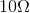resistors are placed in a circuit. What is the difference in equivalent resistance when the resistors in series versus when they are in parallel?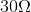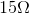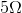Explanation:

In series, the equivalent resistance is equal to the sum of each individual resistance. In parallel, the inverse of equivalent resistance is equal to the sum of the inverses of each individual resistance.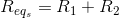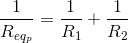We can calculate these two values, and then find the difference between them.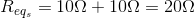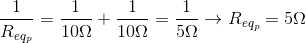Find the difference: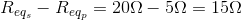### Example Question #4 : Understanding Series And Parallel

Basic Computational

Six resistors are arranged in a series. Their resistances are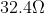,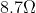,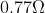,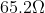,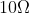, and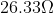. What is their total resistance?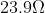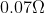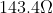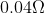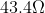Explanation:

When working in a series, the total resistance is the sum of the individual resistances.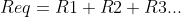Use the given values for each individual resistor to solve for the total resistance.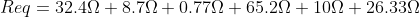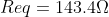### Example Question #5 : Understanding Series And Parallel

What is the total resistance of a parallel circuit with resistors of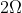,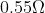, and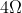?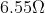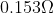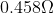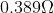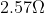Explanation:

The formula for resistance in parallel is: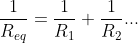We are given the values for each individual resistor, allowing us to solve for the total resistance.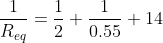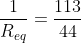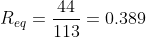### Example Question #6 : Understanding Series And Parallel

Conceptual

When resistors are connected in series

The potential difference across each is the same

More than one of the given answers are true

The same power is dissipated in each one

The current flowing in each is the same

The current flowing in each is the same

Explanation:

In series, there are no branches for the current to travel down.  Therefore it is like a one lane highway so the current is the same throughout the entire circuit.

### Example Question #7 : Understanding Series And Parallel

When resistors are connected in parallel, we can be certain that

Their equivalent resistance is greater than the resistance of any one of the individual resistances

The power dissipated in each is the same

The potential difference across each is the same

The same current flows in each one

The potential difference across each is the same

Explanation:

Consider a set of parallel resistors that are connected to the battery.  Each resistor is on its own loop to the battery.  Therefore each resistor has its own potential difference that is equal to the battery.  That is why if one bulb goes out in a parallel circuit, the other stays lit.  Each resistor has its own connection independent of the others in the circuit.

### Example Question #8 : Understanding Series And Parallel

An electric circuit is set up in series with five resistors. If the resistors remain the same, but the circuit is now set up with the resistors in parallel, how would this affect the total resistance?

Resistance would remain unchanged

Resistance would increase substantially

Resistance would decrease substantially

We need to know the numerical values to solve

Resistance would decrease substantially

Explanation:

Think of resistors as doors, preventing the flow of people (electrons). Imagine the following scenarios: a large group of people are in a room and all try to leave at once. If the five resistors are in series, that's like having all of these people trying to go through all five doors before they can leave. In a circuit, all the electrons in the current must pass through every resistor.

If the resistors are in parallel, it's like having five separate doors from the room. All of a sudden, the group can leave MUCH faster, encountering less resistance to their flow out of the room. The path of the electrons can split, allowing each particle to pass through only one resistor.

From a formula perspective, the resistors in series are simply summed together to find the equivalent resistance.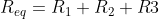In parallel, however, the reciprocals are summed to find the reciprocal equivalent resistance.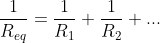Adding whole numbers will always give you a much greater result than adding fractions. For the exact same set of resistors, arrangement in series will have a greater total resistance than arrangement in parallel.

### Example Question #9 : Understanding Series And Parallel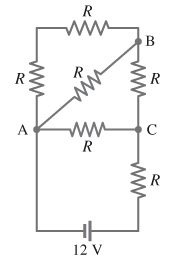What is the net resistance of the circuit connected to the battery?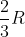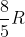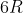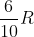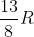Explanation:

To find the net resistance of the circuit, we must summarize all of the different resistors, one piece at a time.  To begin, let’s start with the two resistors in the upper left had corner which are in series.  In series resistance can be added together.  We can replace these two resistors with a single resistor that is valued at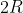.

Next, this new resistor is in parallel with the resistor that is on the diagonal line from point A to point B.  In parallel we can add resistors through the equation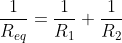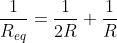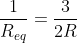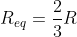This new resistor is now in series with the resistor that is between points B & C.  In series we can just add these resistors up.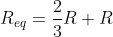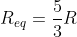This new resistor is now in parallel with the resistor that is between points A & C.  In parallel we can add resistors through the equation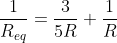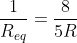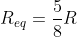This new resistor is now in series with the resistor that is between the battery and point C.  In series we can just add these resistors up.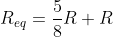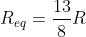### Example Question #10 : Understanding Series And Parallel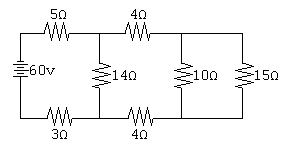What is the net resistance of the circuit connected to the battery?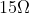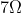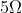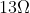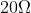Explanation:

To begin, let us start with theresistor and theresistor that are in parallel. In parallel we can add resistors through the equation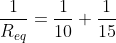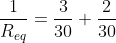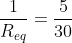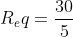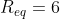This new resistor is now in series with the tworesistors.  In series we can just add these resistors up.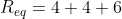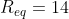This new resistor is now in parallel with the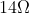resistor. In parallel we can add resistors through the equation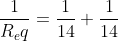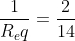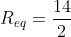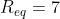This new resistor is now in series with the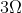andresistor.  In series we can just add these resistors up.